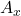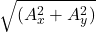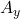## If vector A has components Ax and Ay and makes an angle θ with the +x axis, thena. Ax +Ay (where A is magnitude of A)b. θ= Ay/Axc. Cosθ=

Question

If vector A has components Ax and Ay and makes an angle θ with the +x axis, thena. Ax +Ay (where A is magnitude of A)b. θ= Ay/Axc. Cosθ= Ax/ √ (Ax^2+ Ay^2)d. Tanθ= Ax/Ay

in progress 0
2 months 2021-07-25T05:26:07+00:00 1 Answers 2 views 0

c.  Cos θ =/Explanation:

A vector is any quantity that has both magnitude and direction. The given vector A has componentsand, and makes angle  θ with +x axis.

Thus;

Resultant of the vector, A =Therefore, the components, angle and resultant of vector A can be represented in magnitude and direction by the three sides of a right angled triangle.

Applying the appropriate trigonometric function to the triangle for vector A, we have;

Cos θ =÷⇒   Cos θ =/The correct option is C.